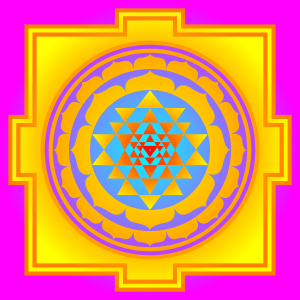Sticky post

We have lost the relationship between Number and Form or Number and Magnitude as the Ancient Greeks called their Forms. A few years ago a Revolution in Mathematics and Physics has started. This revolution is caused by Geometric Algebra. In Geometric Algebra the Ancient Theories of Euclid and Pythagoras are reevaluated. Numbers are Scalar (Quantum) Movements of Geometric Patterns and not Static Symbols of Abstractions that have nothing to do with our Reality. Movements and not Forces are the Essence of Physics. The basic rule Movement = Space/Time (v=s/t) shows that  Time and Space are two Reciprocal 3D-Spaces. Our Senses … Continue reading About Number and MagnitudeSticky post

# About Mystical Number Theory and Pascal’s Triangle

The first part of this Blog is about the Triangular numbers, related to the Number 3, the Holy Trinity. The second part shows that Pascal’s Triangle (called Meru’s Mountain in Mystics), the Binomial Expansion,  contains every Possible Mystical Number Pattern (including the Triangular Numbers) you can Imagine. Pascal Triangle also shows that our Universe is a combinatorial miracle. It explores every possibility, is always in balance, expands and moves back to the beginning which is and was the Void, the Empty Set, the merge of Every Paradox, that is Possible. About Mystical Number-Patterns The Sēpher Yəṣîrâh (Book of Formation or Book … Continue reading About Mystical Number Theory and Pascal’s Triangle